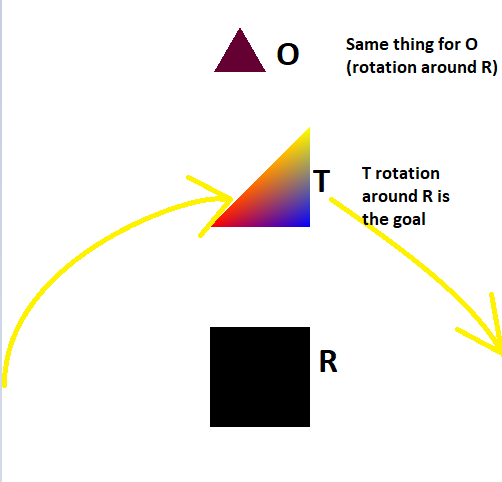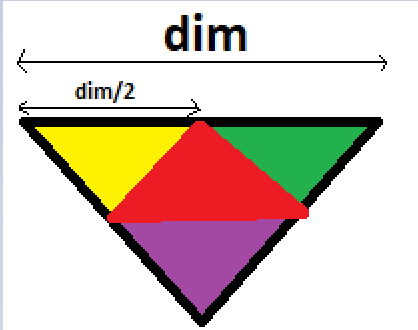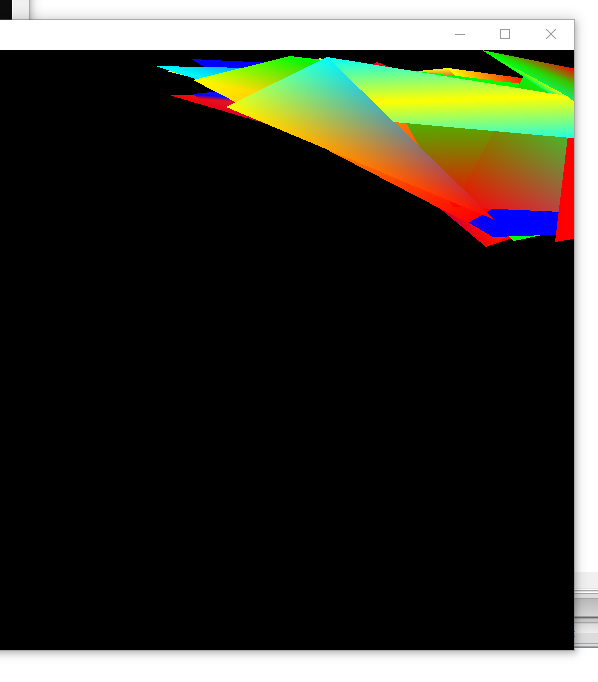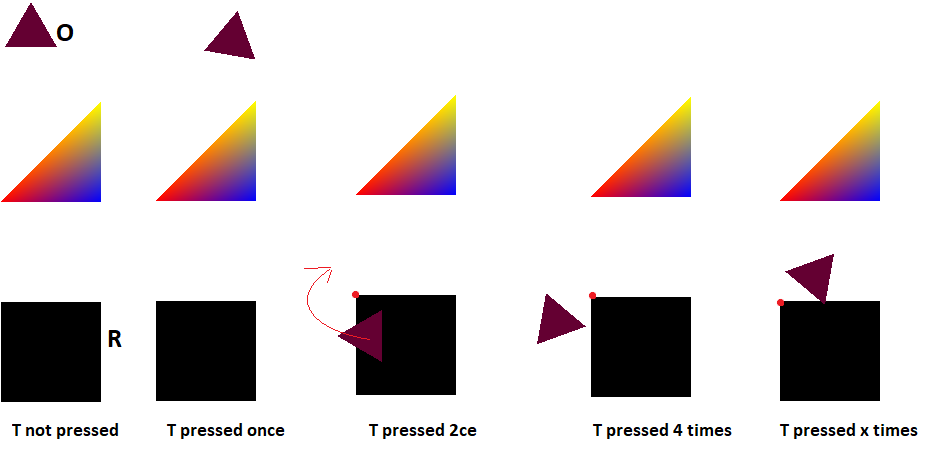###Author Topic: A total of 7 questions  (Read 2258 times)

0 Members and 1 Guest are viewing this topic.

#### Power##### A total of 7 questions
« on: April 01, 2020, 12:10:39 am »
Hello,
I don't want to make a post for every question, maybe i should?

Here are all the questions i encountered so far, if someone could enlighten.. :

1) i reread almost all graphics tutorials except the "view" one, and i did not find a single example of a use of the a Transformable class with a matrix, i saw this on the tutorial page (https://www.sfml-dev.org/tutorials/2.5/graphics-transform.php)
sf::Transform t3(2.f, 0.f, 20.f,
0.f, 1.f, 50.f,
0.f, 0.f, 1.f);
I do understand how a Transform can "absorb" many transformation (Translate, rotate,scale...etc), and then merge the transform in a state or directly on a draw function.
But i have no clue what this matrix means?
I have been advised on another topic to use this matrix to do a rotation :
[cos(A)     -sin(A)]
[sin(A)      cos(A)]

I thought i could understand it later, but after rereading and trying to understand some SFML examples such the "tilemap" i still dont know what matrix have to do with Transformable.

2) ROTATION:
After re reading almost all turorials (except the "View" one) and trying to remember all graphics functions SFML have, i can finally say i dont know well how getRotation works.
Here an example of a rectangular shape with 2 other shapes i want to make them rotate AROUND the rectangle, the three shapes are named R (Rectangle), C(A triangle made with vertices) and O(and Object with a traingular shape, its the smallest and has one color, compared to the C triangle which is multi coloured).sf::RenderWindow window(sf::VideoMode(800, 600), "It Works!", sf::Style::Close, contextSettings);
window.setFramerateLimit(30);

///SHAPE1
sf::RectangleShape R; // rectangle
R.setPosition(450,350);
R.setSize(sf::Vector2f(100,100));
R.setFillColor(sf::Color::Black);
///SHAPE2
sf::VertexArray V(sf::Triangles,3); //Triangle closest to the rectangle
V.position = sf::Vector2f(R.getPosition().x,R.getPosition().y-100); // which is (450,350)
V.position = sf::Vector2f(R.getPosition().x+100,R.getPosition().y-100); // which is (550,350)
V.position = sf::Vector2f(R.getPosition().x+100,R.getPosition().y-200); // which is (550,250)
V.color = sf::Color::Red;
V.color = sf::Color::Blue;
V.color = sf::Color::Yellow;
///SHAPE3
sf::CircleShape O(30,3); // farest shape : object

O.setPosition(R.getPosition().x,R.getPosition().y-300);
O.setFillColor(sf::Color(100,0,50));

//        newOctagon.setOutlineThickness(6.f);
//    newOctagon.setOutlineColor(sf::Color::Red);
O.setOrigin(R.getOrigin());
O.rotate(45);
//R.rotate(45);

// triangle.color = sf::Color::Red;

while (window.isOpen())
{

sf::Event event;
while (window.pollEvent(event))
{
if (event.type == sf::Event::Closed)
window.close();
}

window.clear(sf::Color::White);

window.draw(R);
window.draw(V);
window.draw(O);

window.display();

So is it possible to make O which is a CircleShape, rotate around R?
Is it possible to make T which is a vertex type, rotate around R?

3)
Is it possible to fill multiple colours for regular shapes(not vertices) choosing areas with "Rect" ? (
Example like this :4) On the class sf::Unit8
I am not sure How it it used, because i find no classe called "Unit8" browsing the documentation: here an example i saw somewhere :
sf::Uint8* pixels = new sf::Uint8[width * height * 1]; // * 4 because pixels have 4 components (RGBA)
//sf::Uint8* pixels = new sf::Uint8[width /2];
// sf::Uint8* pixels2 = sf::Vector2f(400,0)...; // get a fresh chunk of pixels (the next frame of a movie, for example)
tex1.update(pixels); /// FIRST PROB

What happens to the Texture tex1 here?

5) A random vertices generator does nor work inside a new "MyEntity" class while drawing it on the window, but the code works outside the new class (inside main), this:
int N;
cout << endl << " choose N : (below 26000) " << endl;
cin >> N;
sf::VertexArray Vexy(sf::TriangleStrip,N);
srand(time(nullptr)); // use current time as seed for random generator
for(int i;i!=N;i++)
{

int random_variable2 = rand()%400+1;
int random_variable3 = rand()%500+300;
Vexy[i].position = sf::Vector2f(random_variable2+random_variable3, random_variable2/2);
Vexy[i].color = colorArray[rand()%5];

}

Result :But while i m using a new classe, like this one, it does not work(does not show up with the function draw)
using namespace std;
class MyEntity : public sf::Drawable, public sf::Transformable
{
public:

MyEntity(float NewPointcount) : PC(NewPointcount)
{
sf::VertexArray Vex(sf::TriangleStrip, PC);
const sf::Color colorArray={sf::Color::Cyan, sf::Color::Blue, sf::Color::Green, sf::Color::Red, sf::Color::Yellow };

///USING THE FUNCTION RAND => HASARD
srand(time(nullptr)); // use current time as seed for random generator
Vex.position = sf::Vector2f(0, 0);
Vex.color = colorArray[rand()%5];
for (int n=1; n != PC-1; ++n)
{
int random_variable = rand()%200+1;
int random_variable3 = rand()%600+300;
cout << "Random value on [0 " << RAND_MAX << "]: "
<< random_variable << '\n';

Vex[n].position = sf::Vector2f(random_variable, random_variable3);
cout << rand()%5 << endl;

Vex[n].color = colorArray[rand()%5];
}
Vex[PC-1].position =  sf::Vector2f(0, 0);
Vex[PC-1].color = colorArray[rand()%5];

};

private:

virtual void draw(sf::RenderTarget& target, sf::RenderStates states) const
{
// apply the entity's transform -- combine it with the one that was passed by the caller
states.transform *= getTransform(); // getTransform() is defined by sf::Transformable

// apply the texture
states.texture = &m_texture;

// you may also override states.shader or states.blendMode if you want

// draw the vertex array
target.draw(m_vertices, states);
}

sf::VertexArray m_vertices;
sf::Texture m_texture;
int PC;
};

and inside the main :
MyEntity ME(6);
..
window.clear(sf::Color::Black);
window.draw(ME);
window.display();

Nothings shows up. Also the difference between using the CLASS and the exemple before is that i set the first and last vertices outside the "for".

6) My last question is about the "Tilemap" example :
Which can be found at the bottom of this page : https://www.sfml-dev.org/tutorials/2.5/graphics-vertex-array.php

I had a hard time  undesrtanding the the variables tu and tv, i think a sentence should be written as comment to describe them, it's a google search on them and made me fall on one of the old posts from SFML forum and made me understand that : "tu and tv are the tile coordinates of the texture. tu is the column of the tile, and tv its line."

Okay what does this line exactly do?
m_vertices.resize(width * height * 4);
I can read here : https://www.sfml-dev.org/documentation/2.5.1/classsf_1_1VertexArray.php
that the resize function purpose is to change the number of "points" or "vertices".
Dont we always need 4-5 vertices to draw a tile? Which is rectangular?
I really dont get this line i keep finding everywhere : "(width * height * 4)"

7) Also, i did an experiment, with adding a "cout" to know the location of the FIRST point of each tile + the location of the first TexCoord for each said point :
quad.position = sf::Vector2f(i * tileSize.x, j * tileSize.y);
cout<< " position 1           :" << i * tileSize.x << "-" << j * tileSize.y << endl;
quad.position = sf::Vector2f((i + 1) * tileSize.x, j * tileSize.y);
quad.position = sf::Vector2f((i + 1) * tileSize.x, (j + 1) * tileSize.y);
quad.position = sf::Vector2f(i * tileSize.x, (j + 1) * tileSize.y);

// define its 4 texture coordinates
quad.texCoords = sf::Vector2f(tu * tileSize.x, tv * tileSize.y);
cout<< " coordinates TEX 1 :" << tu * tileSize.x<< "-" << tv * tileSize.y << endl;
quad.texCoords = sf::Vector2f((tu + 1) * tileSize.x, tv * tileSize.y);
quad.texCoords = sf::Vector2f((tu + 1) * tileSize.x, (tv + 1) * tileSize.y);
quad.texCoords = sf::Vector2f(tu * tileSize.x, (tv + 1) * tileSize.y);

And i noticed this :I still find it hard to understand it, i guess i will have to do many more examples to get it quicker, it seems that quad.position thing browses line by line after finishing a line one after another (from left to right), while quad.texCoors seems to browse vertically (from up to down then it changes the collumn)
Anyway this last question was not really a question.

« Last Edit: April 01, 2020, 12:14:44 am by Power »

#### Hapax

• Hero Member
••• Posts: 3100
• My number of posts is shown in hexadecimal.##### Re: A total of 7 questions
« Reply #1 on: April 01, 2020, 02:36:28 am »
1)
These can explain it much better than I can:
https://en.wikipedia.org/wiki/Matrix_(mathematics)
https://en.wikipedia.org/wiki/Matrix_(mathematics)#Linear_transformations
https://en.wikipedia.org/wiki/Rotation_matrix

2)
Yes, it's possible.
Rotation is always around the object's origin.
You can set O's origin to the offset between O and R and then set O's position to the same as R. O's origin will offset the visual position of O but all rotation will go around R.
Since they (O and R) seem to be 300 apart in your example, O's origin would be (0, 300).

3)
Not sure I understand the question.
You can have different coloured triangles in a single vertex array but not in a standard SFML shape (without using a texture).

4)
sf::Uint8 is an unsigned 8-bit integer.
Your example is an array of those necessary for an image - basically an array of bytes. Each colour component is an 8-bit value - 0-255.
Although, I would suggest that there is an error; it should be multiplied by 4, not 1, for the four components - one byte each.

5)
Have you tried this without the difference? Does it work? Is it the same?

6)
tu and tv are arbitrary values to reference the tile within the texture and are used to set the texture co-ordinates for that tile.
t is for texture. u and v are common co-ordinate variables for texture (XYZ for space, UVW for texture)

In the tilemap example, it uses a vertex array with the quad primitive. Every one of those quad primitive requires four vertices to define its corners. Those vertices don't have to rectangular.

7)
Firstly, your cyan lines are incorrect. They should point to the same place as the red lines.

The "position" is where they are drawn/shown. You can see that the left row are all x = 0 and that y steps up by the height of the tile (i.e. in 32 increments).
The "coordinates TEX" is where in the texture that tile is using. You can see all the tiles that have grass all have the same co-ordinates. Each different tile has a different value (its location in the texture/tileset image).

Hope some of that helpsSelba Ward - SFML drawables
Kairos - Timing Library
Rectangular Boundary Collision - Rectangular SAT Collision

#### Laurent

• Hero Member
••• Posts: 32504##### Re: A total of 7 questions
« Reply #2 on: April 01, 2020, 08:17:55 am »
Quote
I don't want to make a post for every question, maybe i should?
Let's think about it. What's the difference with you opening 7 different posts, and 7 different users opening 1 post each? None, both are fine. Or, imagine that you had 1 problem per month during 7 months, you wouldn't have the silly idea to edit the same post to add them all to it everytime.

On the other hand, mixing unrelated questions in a single post makes it hard to:
- find relevant information when finding your post through the search engine
- read all that stuff (I personally gave up)
- reply to a single question
- follow 7 different discussions mixed together, after some time passes and replies are posted

Definitely the worst idea ever. I don't know why so many people think it's "bad" to open multiple postsLaurent Gomila - SFML developer

#### Power##### Re: A total of 7 questions
« Reply #3 on: April 01, 2020, 11:53:29 am »
Quote
I don't want to make a post for every question, maybe i should?
Let's think about it. What's the difference with you opening 7 different posts, and 7 different users opening 1 post each? None, both are fine. Or, imagine that you had 1 problem per month during 7 months, you wouldn't have the silly idea to edit the same post to add them all to it everytime.

On the other hand, mixing unrelated questions in a single post makes it hard to:
- find relevant information when finding your post through the search engine
- read all that stuff (I personally gave up)
- reply to a single question
- follow 7 different discussions mixed together, after some time passes and replies are posted

Definitely the worst idea ever. I don't know why so many people think it's "bad" to open multiple postsI understand, and i actually think i have found an old post of yours recommanding users to write multiple posts instead of having one and reviving each time .
The reason i found it useful to put many questions in one topic, is because i saw that few points were related (the rotation part, and then the use of matrix on transformable (which is not found in the tutorial)).
If you could read only one thing is why is there a "sf::Uint8" SFML class, hence the "sf::", that cannot be found on this list? https://www.sfml-dev.org/documentation/2.5.1/annotated.php (it would have been useful for me or other "new comers".
Will try to make separate posts whenever the questions are not related.

1)
These can explain it much better than I can:
https://en.wikipedia.org/wiki/Matrix_(mathematics)
https://en.wikipedia.org/wiki/Matrix_(mathematics)#Linear_transformations
https://en.wikipedia.org/wiki/Rotation_matrix
Oh i see so that's what i was missing. Ok so let's say i have understood the effect of each rotation matrix/linear transformation, how could i use it then? Let's i have this one :
[-1 0]
[0 1]
Which is a Reflection through the vertical axis or wichever matrix containing cos and sin, should i read more to know how to use them? i can't find an example of a code using a matrix transformation (puting aside which matrix you are using, just how can it be used once you got your matrix).

Quote
2)
Yes, it's possible.
Rotation is always around the object's origin.
You can set O's origin to the offset between O and R and then set O's position to the same as R. O's origin will offset the visual position of O but all rotation will go around R.
Since they (O and R) seem to be 300 apart in your example, O's origin would be (0, 300).
Oh delightful, it seems to be working :
..
int rot=10;
..
if(event.key.code==sf::Keyboard::T)
{
O.setOrigin(R.getPosition()-O.getPosition());
O.setPosition(R.getPosition());
O.rotate(rot%360);
rot=rot+10;
}
..
..
window.draw(R);
window.draw(V);
window.draw(O);
...

Result :Note : Only at the second rotation, that O makes it close to R
Note 2 : it seems to be rotating around the origin of R indeed, and not around the center of R, i am pretty sure that fixable.

Quote
3)
Not sure I understand the question.
You can have different coloured triangles in a single vertex array but not in a standard SFML shape (without using a texture).
Understood.
Quote
4)
sf::Uint8 is an unsigned 8-bit integer.
Your example is an array of those necessary for an image - basically an array of bytes. Each colour component is an 8-bit value - 0-255.
Although, I would suggest that there is an error; it should be multiplied by 4, not 1, for the four components - one byte each.
Oh i see so the the Uint8 Pixels variable is an array containing the values for each pixel of the image taking in count all four componenots, meaning if i had a coloured image with a size of (4,5) that would mean the Uint8 variable would be an array of 20x4 length.
So now,  i guess it's time to change every pixel with some kind of "for" and browse the pixels you want to change, one by one or a range by range..?
And then at the end do :
tex1.update(pixels);

?

Would be great to see an example of this.

Quote
5)
Have you tried this without the difference? Does it work? Is it the same?
Yes i just did:
MyEntity(float NewPointcount) : PC(NewPointcount)
{
sf::VertexArray Vex(sf::TriangleStrip, PC);
const sf::Color colorArray={sf::Color::Cyan, sf::Color::Blue, sf::Color::Green, sf::Color::Red, sf::Color::Yellow };

srand(time(nullptr)); // use current time as seed for random generator

for (int n=0; n != PC; ++n)
{

int random_variable2 = rand()%400+1;
int random_variable3 = rand()%500+300;
Vex[n].position = sf::Vector2f(random_variable2+random_variable3, random_variable2/2);

Vex[n].color = colorArray[rand()%5];
}

};

private:

virtual void draw(sf::RenderTarget& target, sf::RenderStates states) const
{
// apply the entity's transform -- combine it with the one that was passed by the caller
states.transform *= getTransform(); // getTransform() is defined by sf::Transformable

// apply the texture
states.texture = &m_texture;

// you may also override states.shader or states.blendMode if you want

// draw the vertex array
target.draw(m_vertices, states);
}

sf::VertexArray m_vertices;
sf::Texture m_texture;
int PC;
};
and
MyEntity ME(6);
..
..
window..draw(ME);
window.display();

Nothing.
Reminder here is the code that works well in the same main :
int N;
cout << endl << " choose N : (below 26000) " << endl;
cin >> N;
sf::VertexArray Vexy(sf::TriangleStrip,N);
srand(time(nullptr)); // use current time as seed for random generator
for(int i;i!=N;i++)
{

int random_variable2 = rand()%400+1;
int random_variable3 = rand()%500+300;
Vexy[i].position = sf::Vector2f(random_variable2+random_variable3, random_variable2/2);
Vexy[i].color = colorArray[rand()%5];

}

...
window.draw(Vexy);

Quote
6)
tu and tv are arbitrary values to reference the tile within the texture and are used to set the texture co-ordinates for that tile.
t is for texture. u and v are common co-ordinate variables for texture (XYZ for space, UVW for texture)

In the tilemap example, it uses a vertex array with the quad primitive. Every one of those quad primitive requires four vertices to define its corners. Those vertices don't have to rectangular.
Those vertices don't have to BE rectangular. You meant? i Guess.

Quote
7)
Firstly, your cyan lines are incorrect. They should point to the same place as the red lines.

The "position" is where they are drawn/shown. You can see that the left row are all x = 0 and that y steps up by the height of the tile (i.e. in 32 increments).
The "coordinates TEX" is where in the texture that tile is using. You can see all the tiles that have grass all have the same co-ordinates. Each different tile has a different value (its location in the texture/tileset image).

Hope some of that helpsAh indeed you got me there, it's a great example, i enjoyed trying to understand it, being new, yeah that was my mistake.
Yes Your help is MUCH APPRECIATED. Thank you so much.

« Last Edit: April 01, 2020, 12:00:03 pm by Power »

#### Laurent

• Hero Member
••• Posts: 32504##### Re: A total of 7 questions
« Reply #4 on: April 01, 2020, 02:21:50 pm »
Quote
If you could read only one thing is why is there a "sf::Uint8" SFML class, hence the "sf::", that cannot be found on this list?
It's not a class, it's an alias (typedef) to a 8-bit unsigned integer type (most likely unsigned char). It exists because SFML hasn't switched yet to C++11 and its std::uint8_t type.

Of course, questions related to the same problem can belong to the same postLaurent Gomila - SFML developer

#### Hapax

• Hero Member
••• Posts: 3100
• My number of posts is shown in hexadecimal.##### Re: A total of 7 questions
« Reply #5 on: April 01, 2020, 03:59:49 pm »
1)
I googled "c++ rotation matrix 2d" and got this:
https://www.geeksforgeeks.org/2d-transformation-rotation-objects/2)
One important thing I may not have been clear about is that you should set the origin of O once - from its original position. After that, it will rotate in a circular motion. It's origin should always be (0, 300), for example.
If you want to rotate around the centre of the rectangle, you can set O's origin to the centre of the rectangle instead of its top-left, or you can change the rectangle's origin to its centre.

4)
4 bytes per pixels.
It may just be easier to use getPixel and setPixel in a sf::Image, which is basically just one of those pixel arrays.

5)
You don't seem to be applying a texture in the one that works.

6)
Yes. My bad. Typing errorSelba Ward - SFML drawables
Kairos - Timing Library
Rectangular Boundary Collision - Rectangular SAT Collision